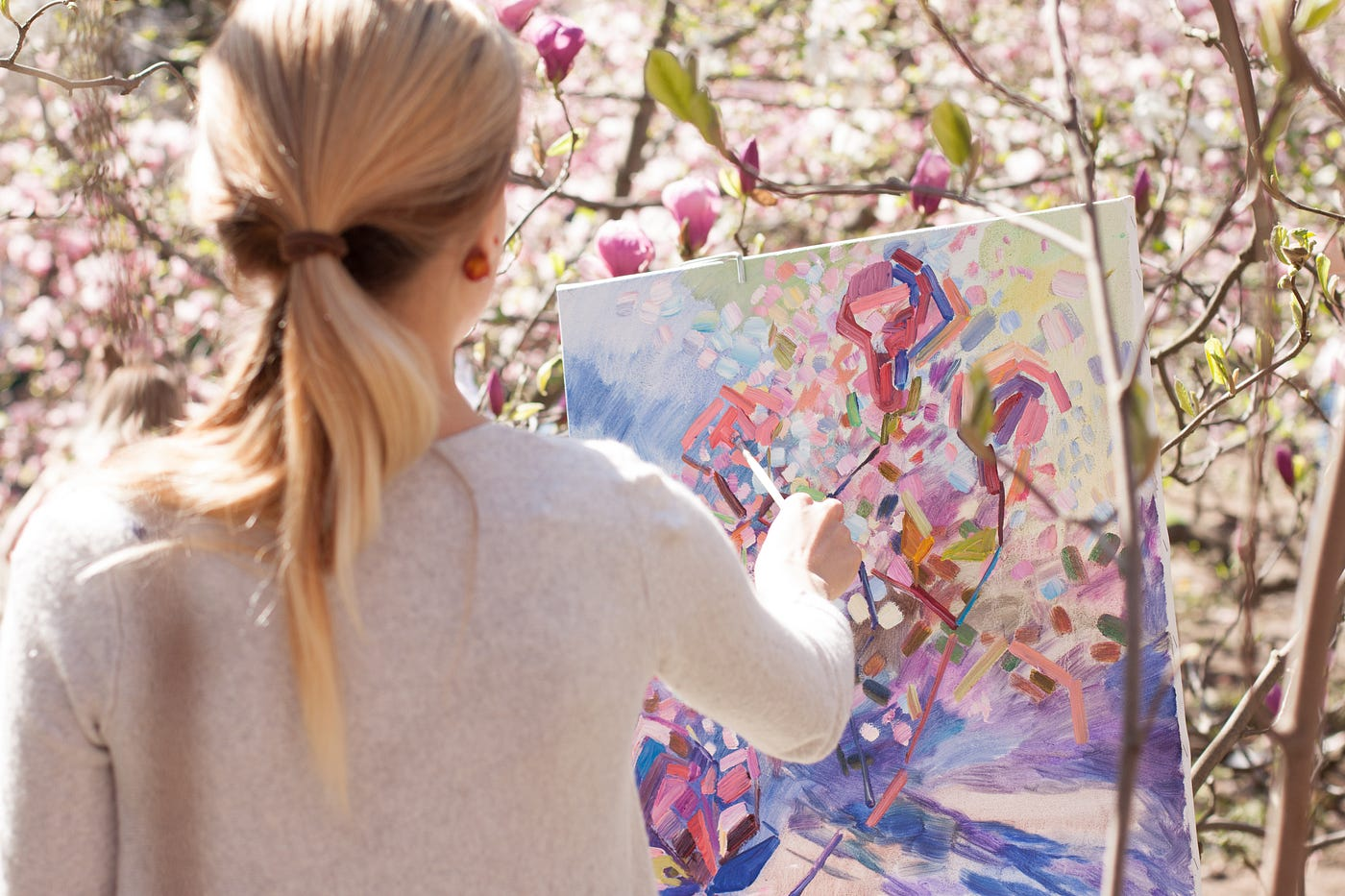# Jetpack Compose — Drawing custom shapes (Circle, Rect, Paths)Photo by Tetiana SHYSHKINA on Unsplash

# Circle

The most basic way to draw the circle

`@Composablefun MyCircle(){    Canvas(modifier = Modifier.size(100.dp), onDraw = {        drawCircle(color = Color.Red)    })}`
`@Composablefun MyMouse(){    Canvas(modifier = Modifier.size(100.dp), onDraw = {        drawCircle(Color.Black, radius = 70f, center = Offset(size.width / 2f, size.height / 2f))        drawCircle(Color.Black, radius = 50f, center = Offset(size.width * 0.25f, size.height * 0.25f))        drawCircle(Color.Black, radius = 50f, center = Offset(size.width * 0.75f, size.height * 0.25f))    })}`

# Rectangle

In the circle example above you see I use size width/height and calculate based on that. You can use float values directly, but you need to multiply by density. Each phone may have a different pixel density. So 100f x 100f may not be on where you expected it to be once its drawn.

`@Composablefun MyRect(){    val density = AmbientDensity.current.density    val w = remember { 100f * density }    val h = remember { 33f * density }    Canvas(modifier = Modifier.size(100.dp), onDraw = {        drawRect(color = Color.Black, size = Size(w, h), topLeft = Offset.Zero)        drawRect(color = Color.Red, size = Size(w, h), topLeft = Offset(0f, h))        drawRect(color = Color.Yellow, size = Size(w, h), topLeft = Offset(0f, h * 2))    })}`

# Paths

Paths allows you to make any custom shape. You can make circles or rectangles if you like. But most of the time you will use Paths to draw triangles, stars, …

`fun MyTriangle(){    val density = AmbientDensity.current.density    val path = Path().apply {        moveTo(50f * density, 0f)        lineTo(100f * density, 100f * density)        lineTo(0f, 100f * density)        close()    }    Canvas(modifier = Modifier.size(100.dp), onDraw = {        drawPath(path, Color.Blue)    })}`

# Star trek logo

This one has a little curve at the bottom and a border. To achive this I used Surface composable and applied the shape on it. The result is pretty nice.

`@Composablefun MyStarTrek(){    val myStarTrekShape = GenericShape { size ->        moveTo(size.width / 2f, 0f)        lineTo(size.width, size.height)        quadraticBezierTo(            size.width * 0.6f,            size.height * 0.4f,            0f,            size.height        )        close()    }    Surface(        shape = myStarTrekShape,        color = Color.Yellow,        border = BorderStroke(3.dp, Color.Black),        modifier = Modifier.size(100.dp)    ) { }}`

--

--